Скачать презентацию C Programming From Problem Analysis to Program Design

f96d7e82368c37937117531e6a46c365.ppt

• Количество слайдов: 48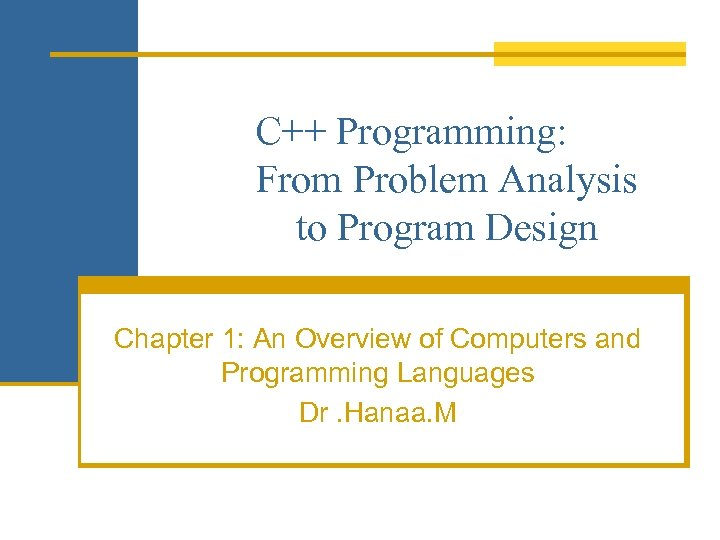C++ Programming: From Problem Analysis to Program Design Chapter 1: An Overview of Computers and Programming Languages Dr. Hanaa. M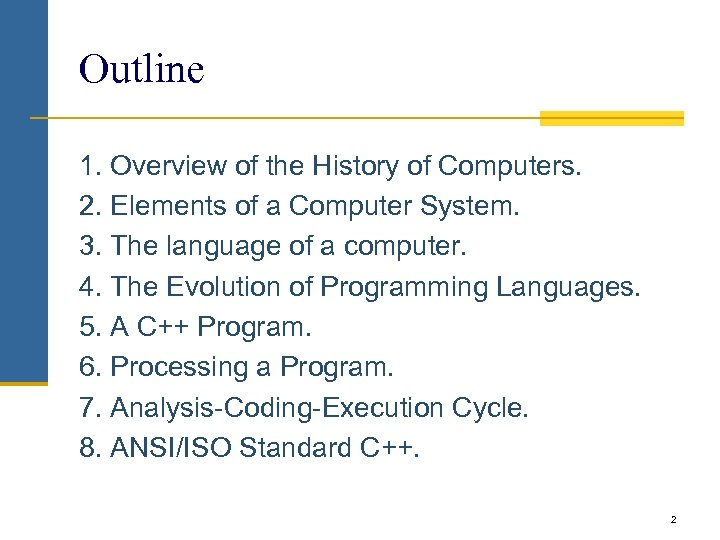Outline 1. Overview of the History of Computers. 2. Elements of a Computer System. 3. The language of a computer. 4. The Evolution of Programming Languages. 5. A C++ Program. 6. Processing a Program. 7. Analysis-Coding-Execution Cycle. 8. ANSI/ISO Standard C++. 2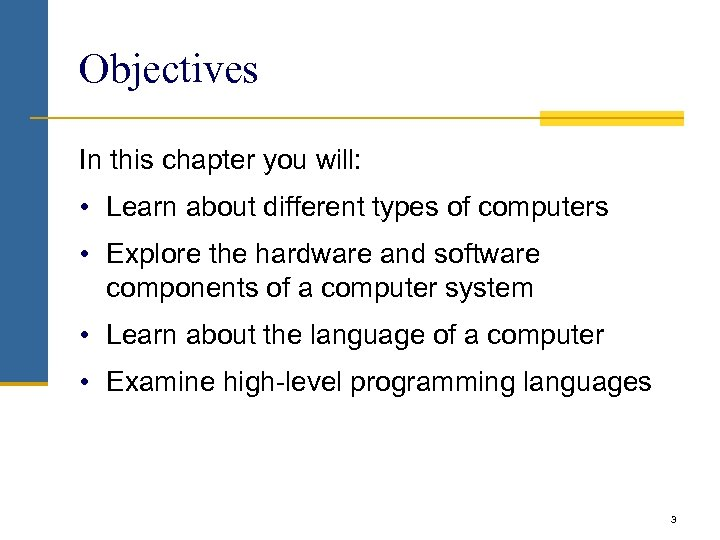Objectives In this chapter you will: • Learn about different types of computers • Explore the hardware and software components of a computer system • Learn about the language of a computer • Examine high-level programming languages 3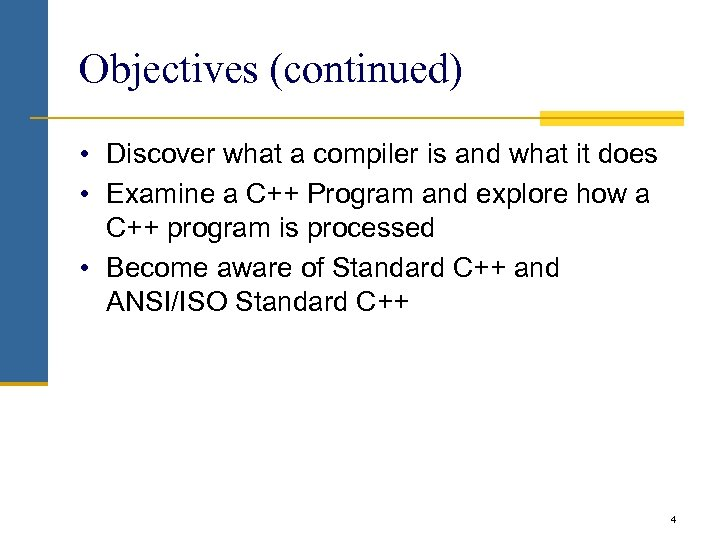Objectives (continued) • Discover what a compiler is and what it does • Examine a C++ Program and explore how a C++ program is processed • Become aware of Standard C++ and ANSI/ISO Standard C++ 4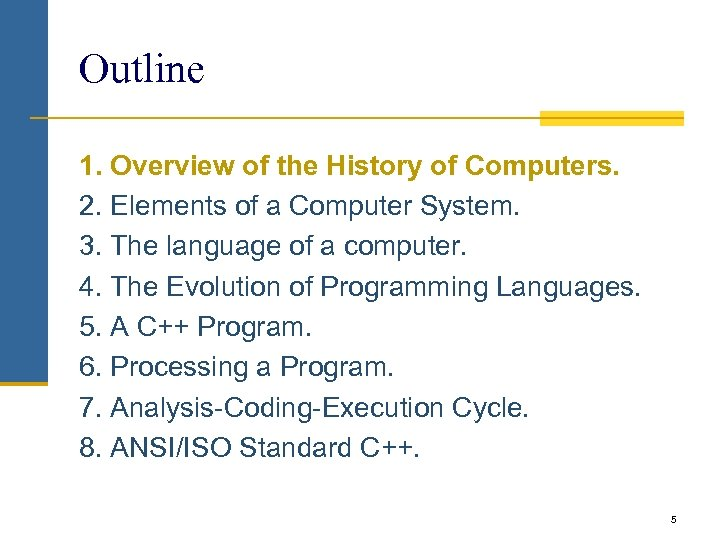Outline 1. Overview of the History of Computers. 2. Elements of a Computer System. 3. The language of a computer. 4. The Evolution of Programming Languages. 5. A C++ Program. 6. Processing a Program. 7. Analysis-Coding-Execution Cycle. 8. ANSI/ISO Standard C++. 5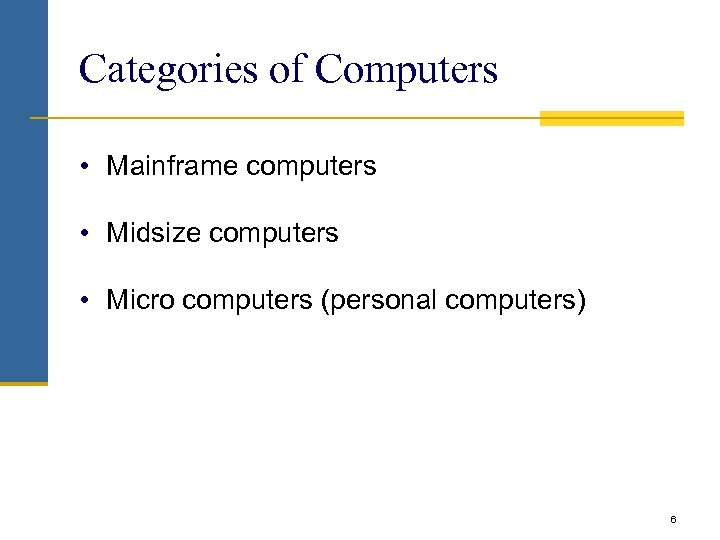Categories of Computers • Mainframe computers • Midsize computers • Micro computers (personal computers) 6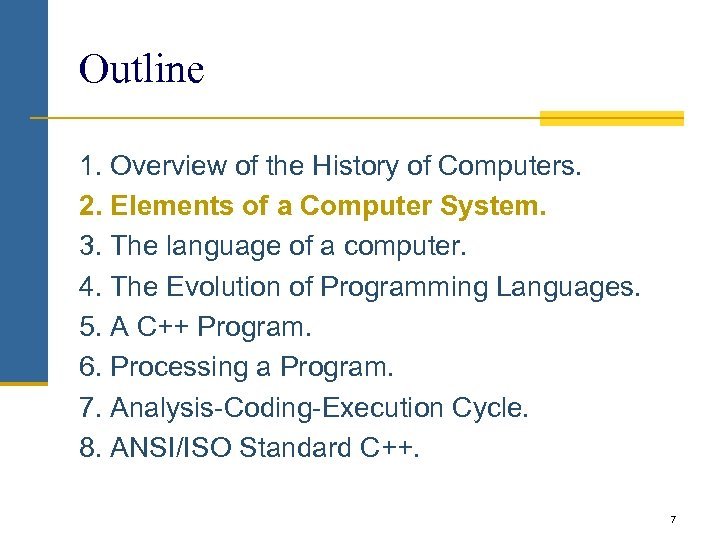Outline 1. Overview of the History of Computers. 2. Elements of a Computer System. 3. The language of a computer. 4. The Evolution of Programming Languages. 5. A C++ Program. 6. Processing a Program. 7. Analysis-Coding-Execution Cycle. 8. ANSI/ISO Standard C++. 7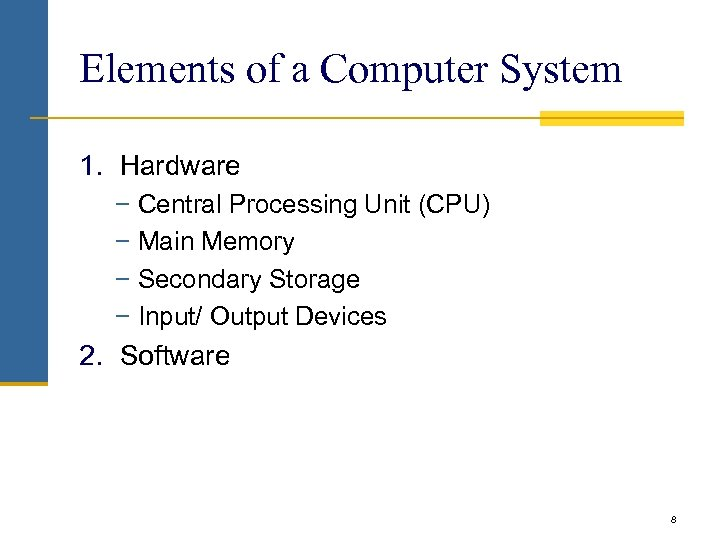Elements of a Computer System 1. Hardware − Central Processing Unit (CPU) − Main Memory − Secondary Storage − Input/ Output Devices 2. Software 8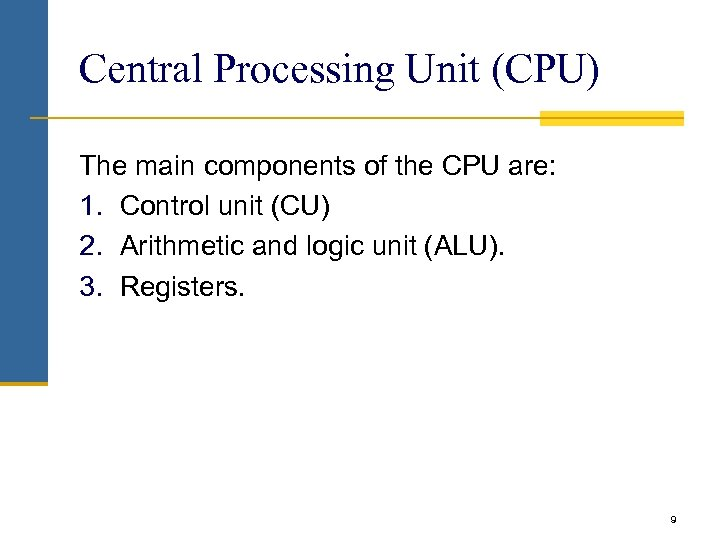Central Processing Unit (CPU) The main components of the CPU are: 1. Control unit (CU) 2. Arithmetic and logic unit (ALU). 3. Registers. 9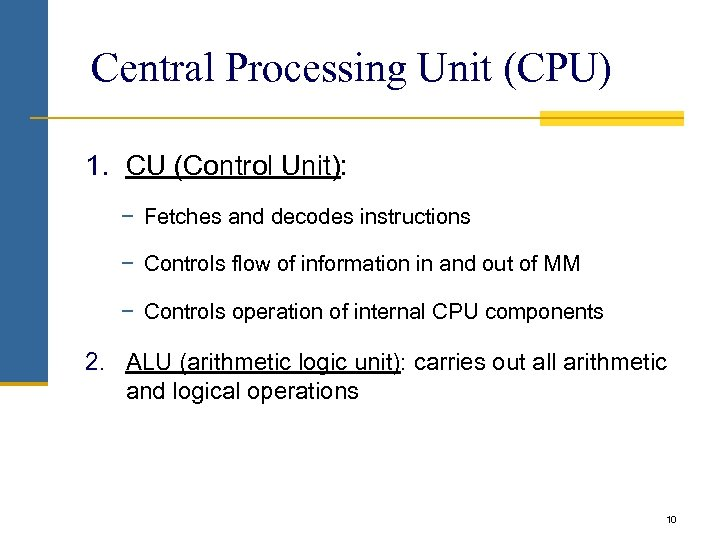Central Processing Unit (CPU) 1. CU (Control Unit): − Fetches and decodes instructions − Controls flow of information in and out of MM − Controls operation of internal CPU components 2. ALU (arithmetic logic unit): carries out all arithmetic and logical operations 10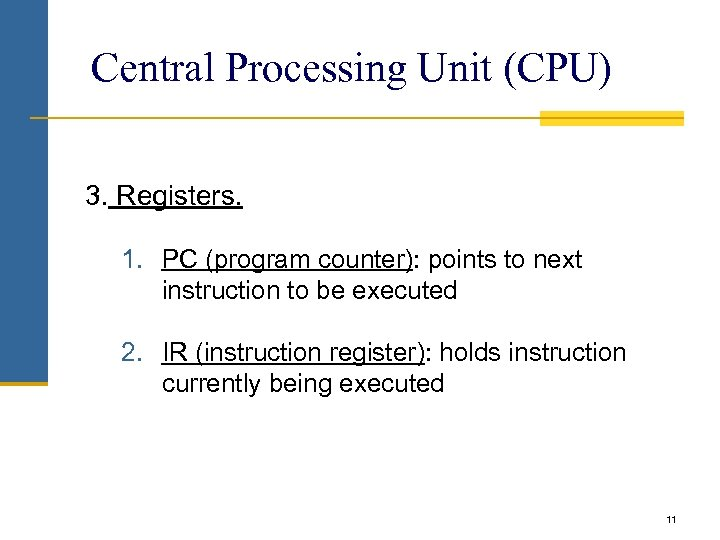Central Processing Unit (CPU) 3. Registers. 1. PC (program counter): points to next instruction to be executed 2. IR (instruction register): holds instruction currently being executed 11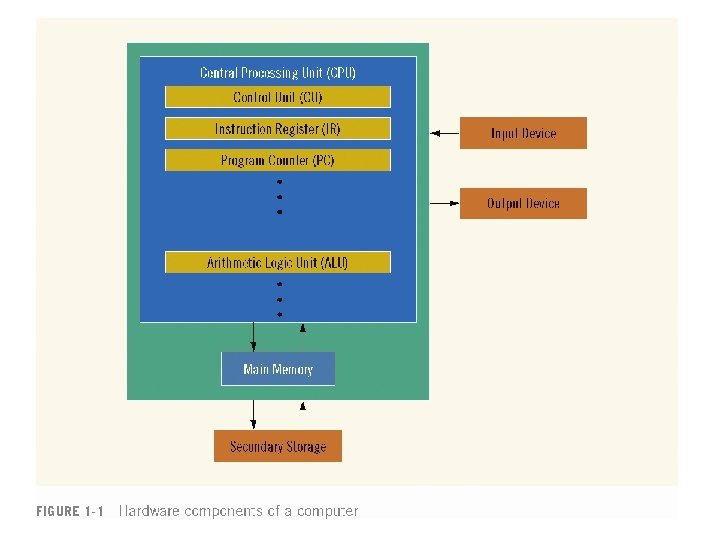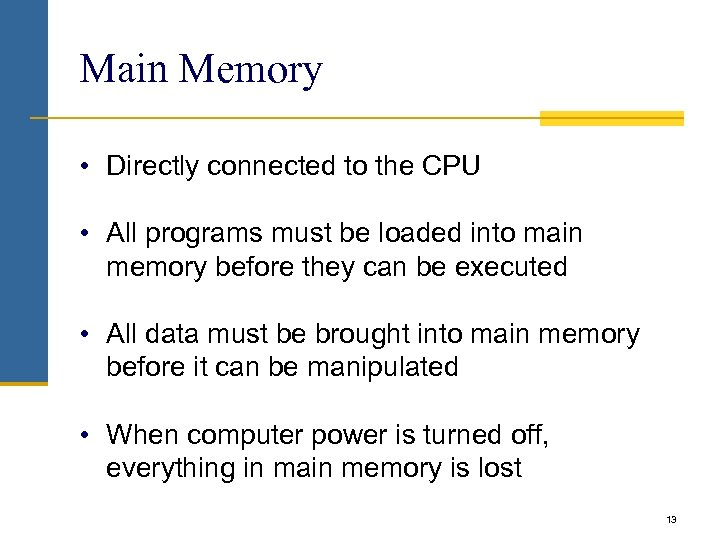Main Memory • Directly connected to the CPU • All programs must be loaded into main memory before they can be executed • All data must be brought into main memory before it can be manipulated • When computer power is turned off, everything in main memory is lost 13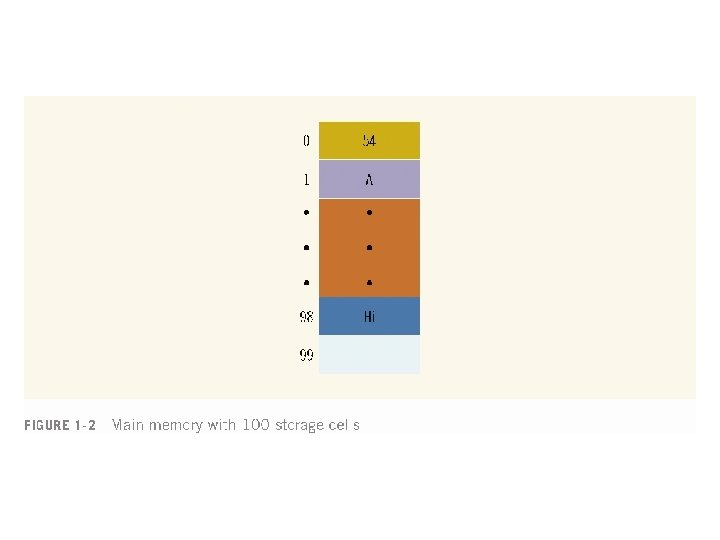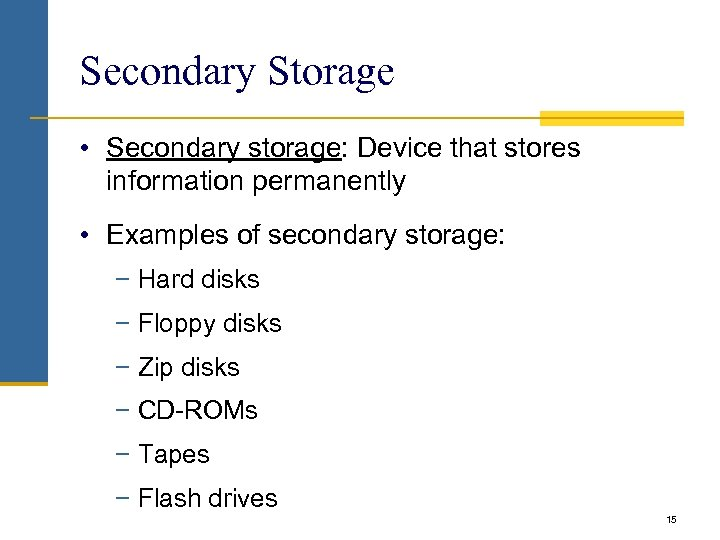Secondary Storage • Secondary storage: Device that stores information permanently • Examples of secondary storage: − Hard disks − Floppy disks − Zip disks − CD-ROMs − Tapes − Flash drives 15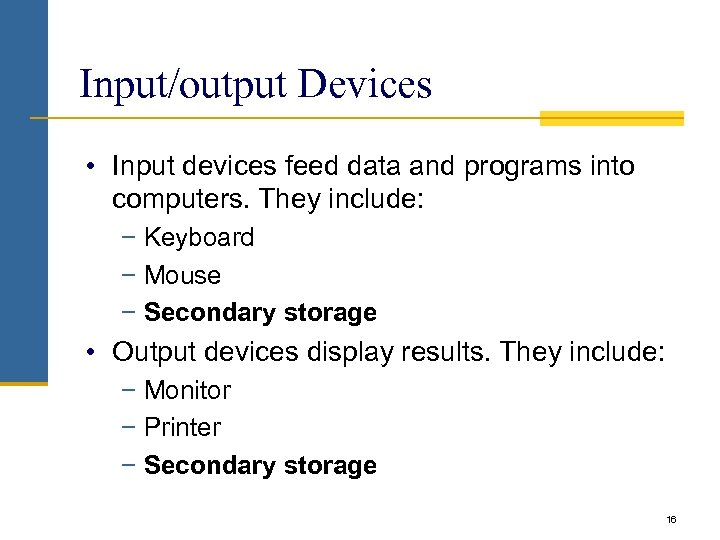Input/output Devices • Input devices feed data and programs into computers. They include: − Keyboard − Mouse − Secondary storage • Output devices display results. They include: − Monitor − Printer − Secondary storage 16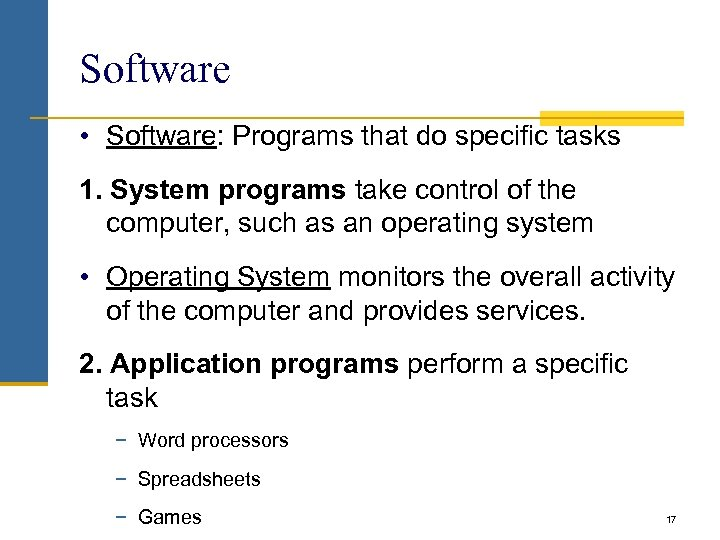Software • Software: Programs that do specific tasks 1. System programs take control of the computer, such as an operating system • Operating System monitors the overall activity of the computer and provides services. 2. Application programs perform a specific task − Word processors − Spreadsheets − Games 17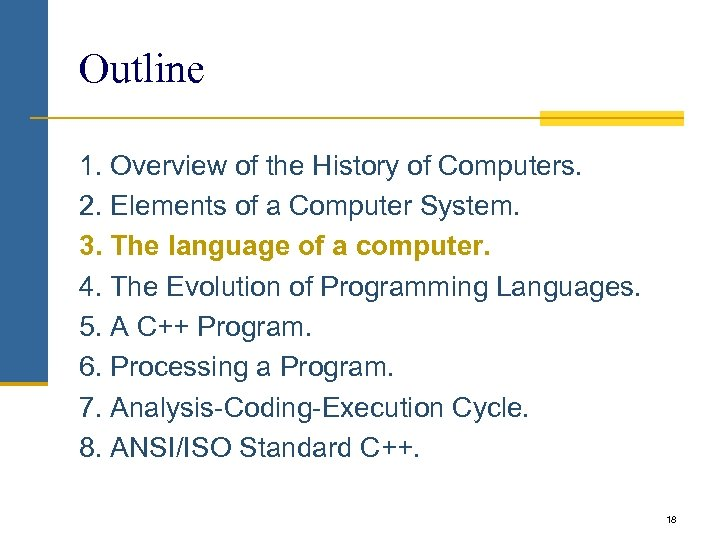Outline 1. Overview of the History of Computers. 2. Elements of a Computer System. 3. The language of a computer. 4. The Evolution of Programming Languages. 5. A C++ Program. 6. Processing a Program. 7. Analysis-Coding-Execution Cycle. 8. ANSI/ISO Standard C++. 18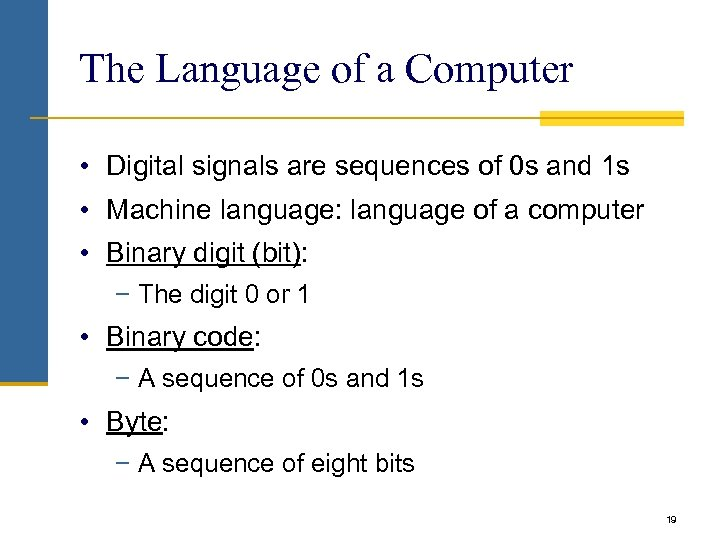The Language of a Computer • Digital signals are sequences of 0 s and 1 s • Machine language: language of a computer • Binary digit (bit): − The digit 0 or 1 • Binary code: − A sequence of 0 s and 1 s • Byte: − A sequence of eight bits 19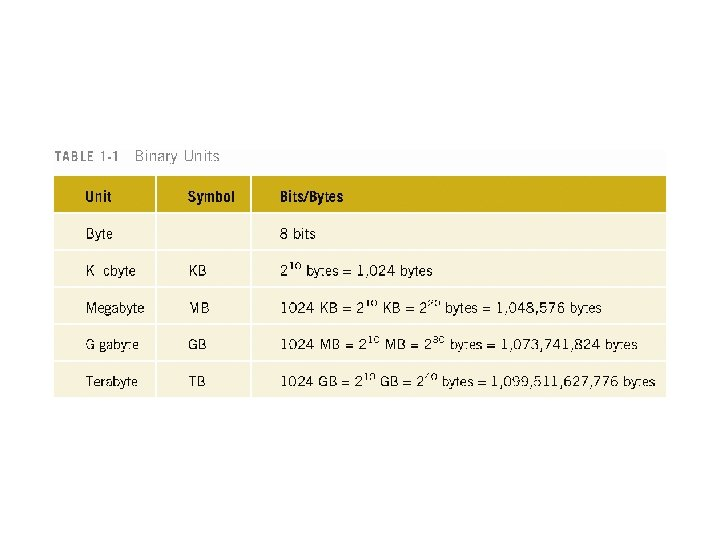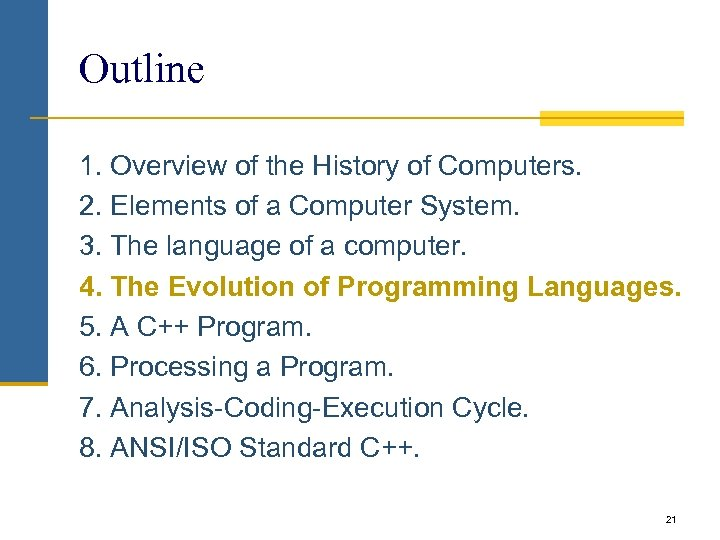Outline 1. Overview of the History of Computers. 2. Elements of a Computer System. 3. The language of a computer. 4. The Evolution of Programming Languages. 5. A C++ Program. 6. Processing a Program. 7. Analysis-Coding-Execution Cycle. 8. ANSI/ISO Standard C++. 21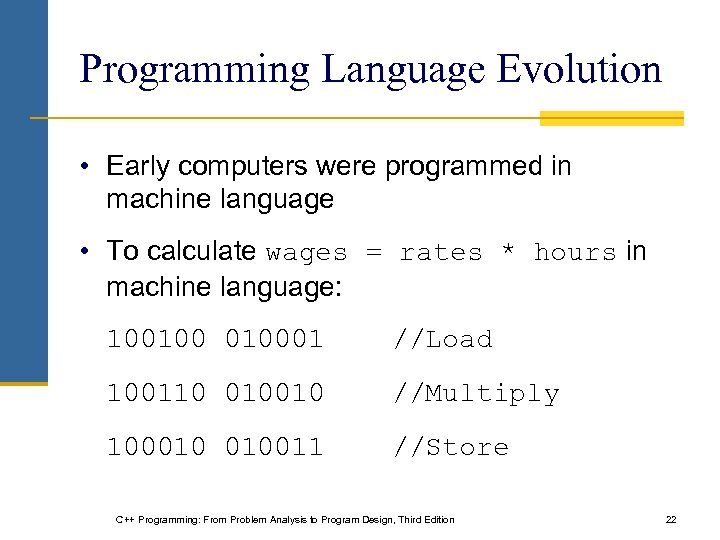Programming Language Evolution • Early computers were programmed in machine language • To calculate wages = rates * hours in machine language: 10010001 //Load 100110 010010 //Multiply 100010 010011 //Store C++ Programming: From Problem Analysis to Program Design, Third Edition 22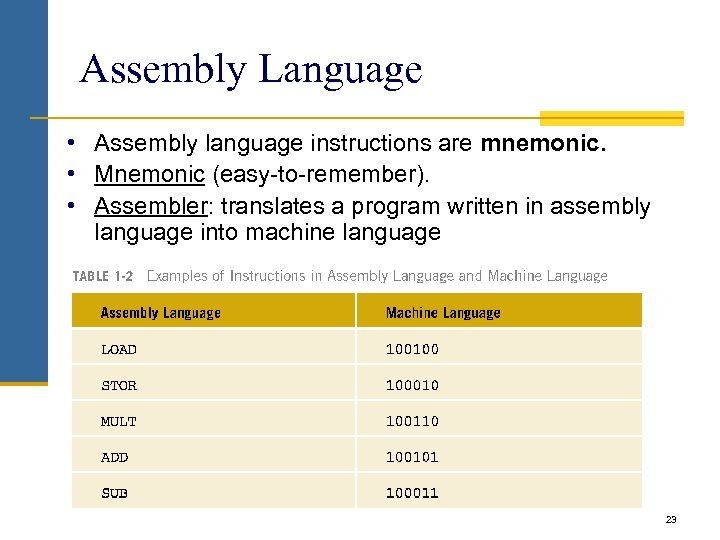Assembly Language • Assembly language instructions are mnemonic. • Mnemonic (easy-to-remember). • Assembler: translates a program written in assembly language into machine language 23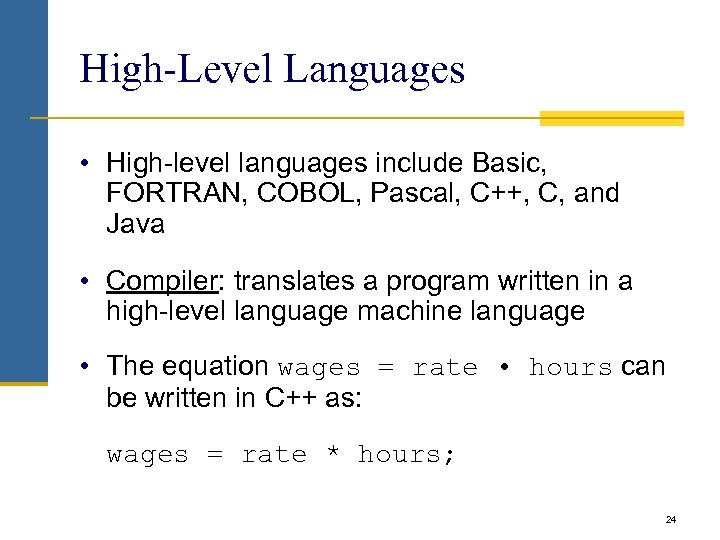High-Level Languages • High-level languages include Basic, FORTRAN, COBOL, Pascal, C++, C, and Java • Compiler: translates a program written in a high-level language machine language • The equation wages = rate • hours can be written in C++ as: wages = rate * hours; 24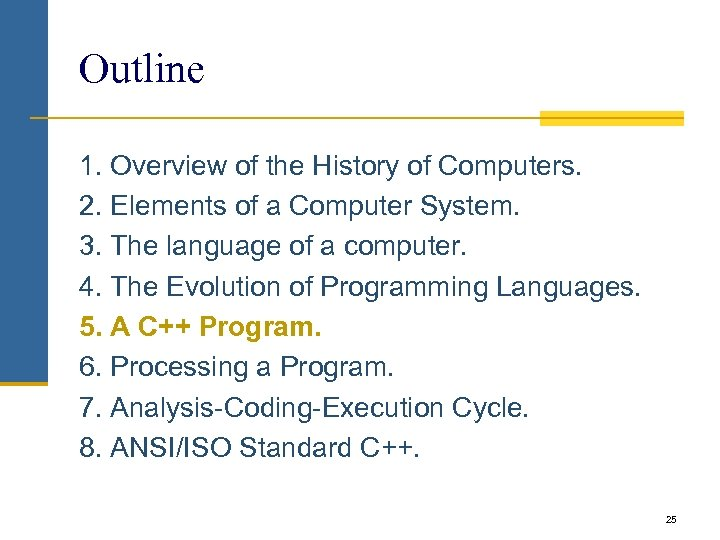Outline 1. Overview of the History of Computers. 2. Elements of a Computer System. 3. The language of a computer. 4. The Evolution of Programming Languages. 5. A C++ Program. 6. Processing a Program. 7. Analysis-Coding-Execution Cycle. 8. ANSI/ISO Standard C++. 25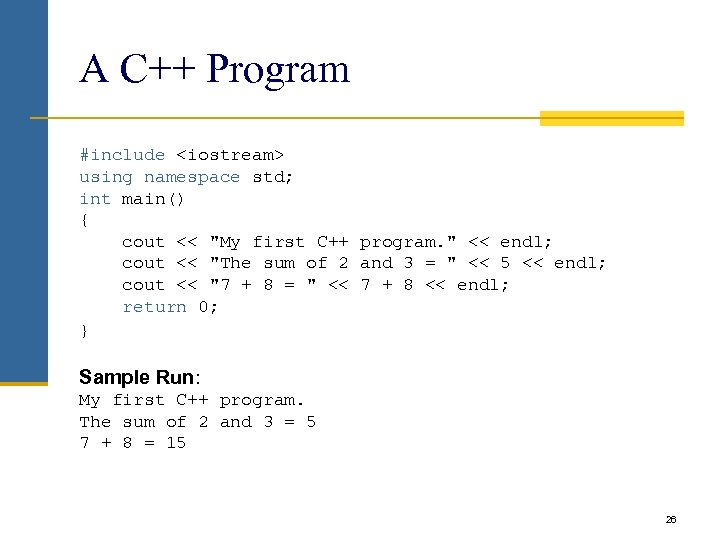A C++ Program #include using namespace std; int main() { cout << "My first C++ program. " << endl; cout << "The sum of 2 and 3 = " << 5 << endl; cout << "7 + 8 = " << 7 + 8 << endl; return 0; } Sample Run: My first C++ program. The sum of 2 and 3 = 5 7 + 8 = 15 26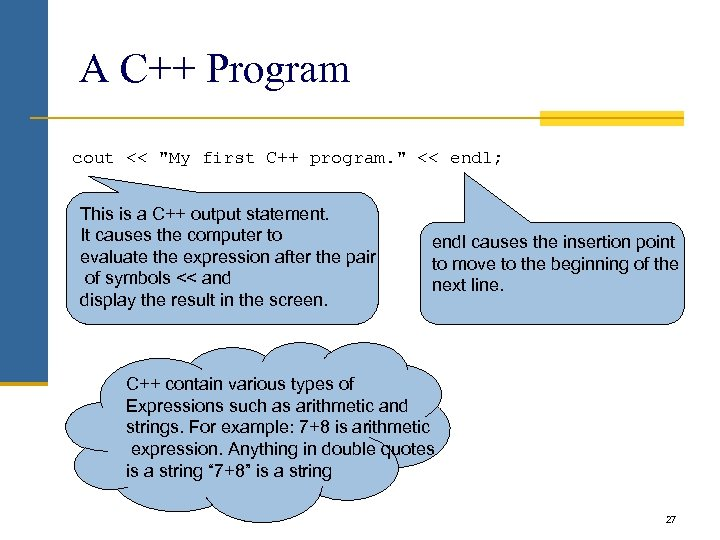A C++ Program cout << "My first C++ program. " << endl; This is a C++ output statement. It causes the computer to evaluate the expression after the pair of symbols << and display the result in the screen. endl causes the insertion point to move to the beginning of the next line. C++ contain various types of Expressions such as arithmetic and strings. For example: 7+8 is arithmetic expression. Anything in double quotes is a string “ 7+8” is a string 27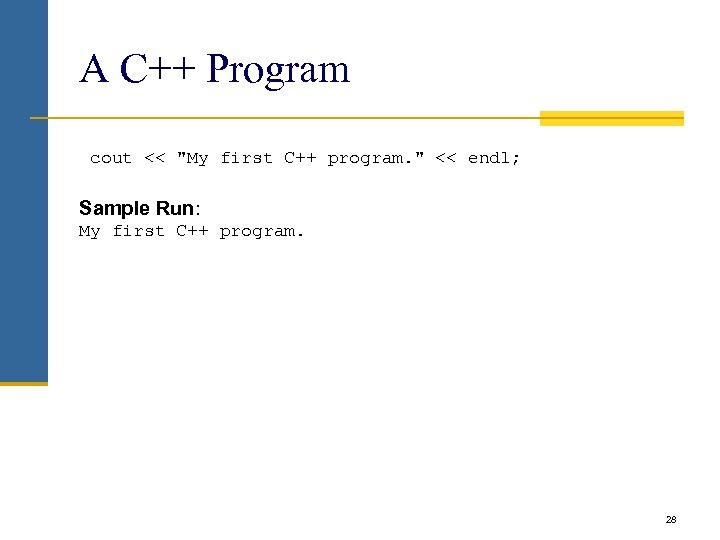A C++ Program cout << "My first C++ program. " << endl; Sample Run: My first C++ program. 28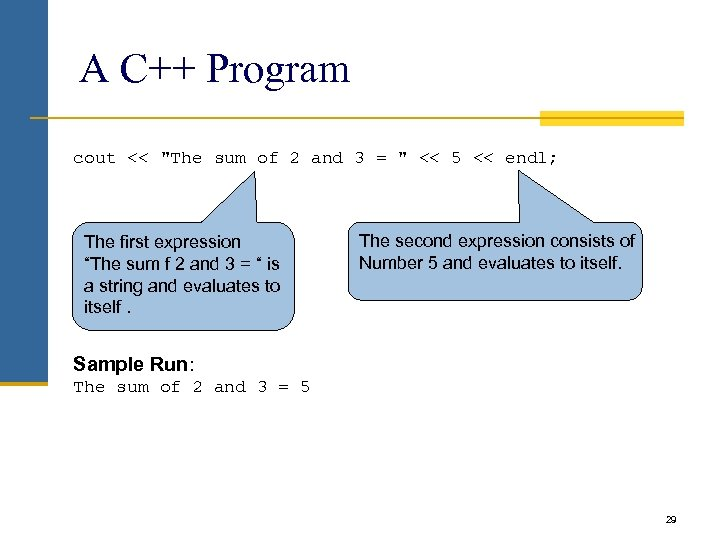A C++ Program cout << "The sum of 2 and 3 = " << 5 << endl; The first expression “The sum f 2 and 3 = “ is a string and evaluates to itself. The second expression consists of Number 5 and evaluates to itself. Sample Run: The sum of 2 and 3 = 5 29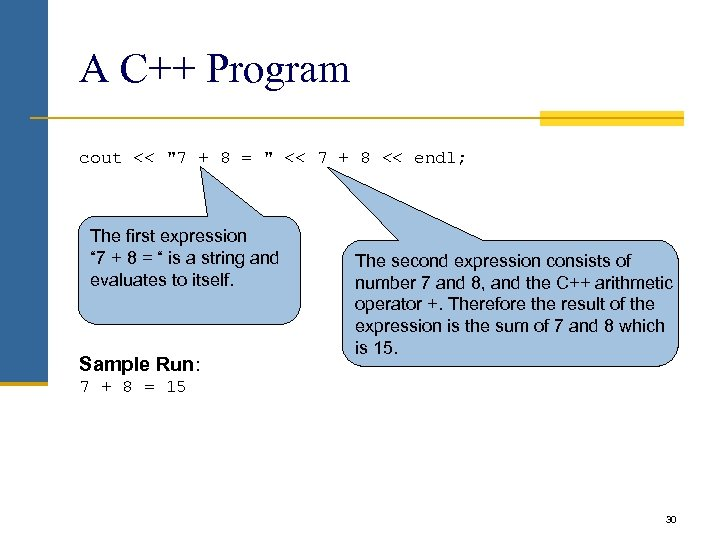A C++ Program cout << "7 + 8 = " << 7 + 8 << endl; The first expression “ 7 + 8 = “ is a string and evaluates to itself. Sample Run: The second expression consists of number 7 and 8, and the C++ arithmetic operator +. Therefore the result of the expression is the sum of 7 and 8 which is 15. 7 + 8 = 15 30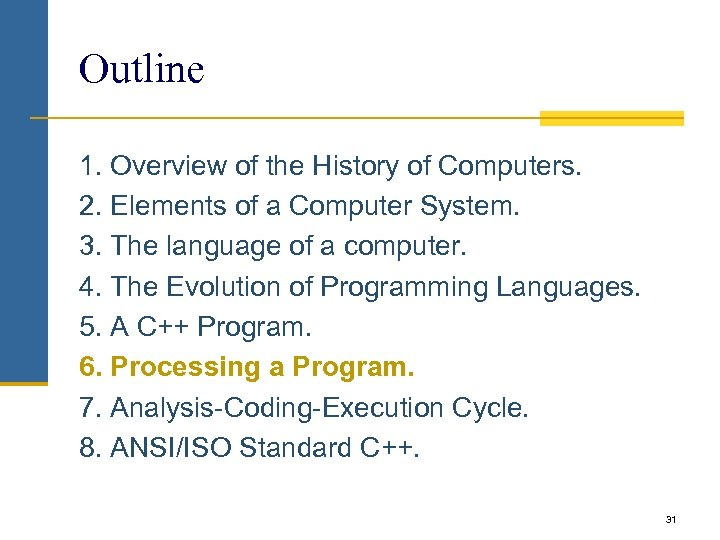Outline 1. Overview of the History of Computers. 2. Elements of a Computer System. 3. The language of a computer. 4. The Evolution of Programming Languages. 5. A C++ Program. 6. Processing a Program. 7. Analysis-Coding-Execution Cycle. 8. ANSI/ISO Standard C++. 31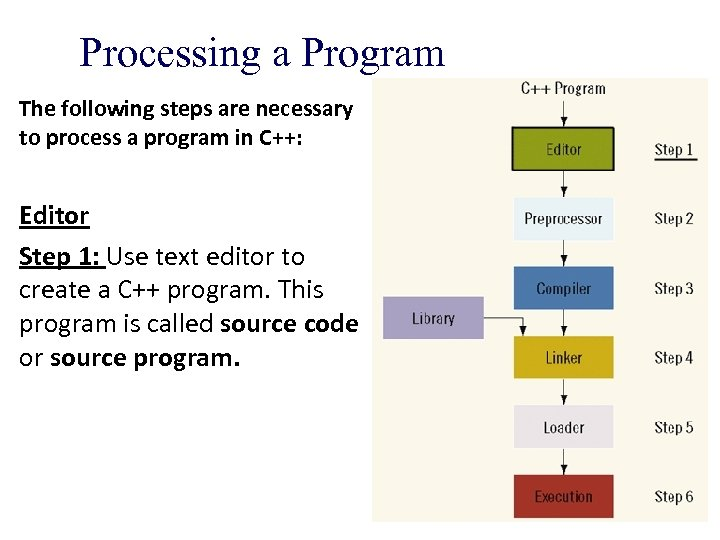Processing a Program The following steps are necessary to process a program in C++: Editor Step 1: Use text editor to create a C++ program. This program is called source code or source program.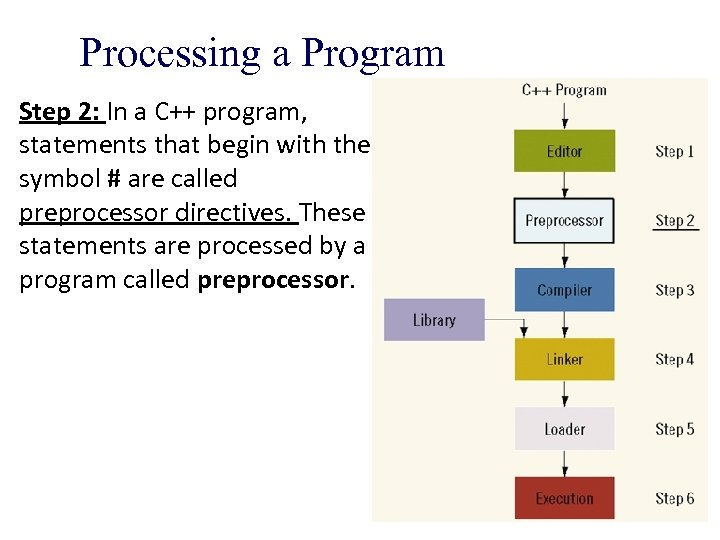Processing a Program Step 2: In a C++ program, statements that begin with the symbol # are called preprocessor directives. These statements are processed by a program called preprocessor.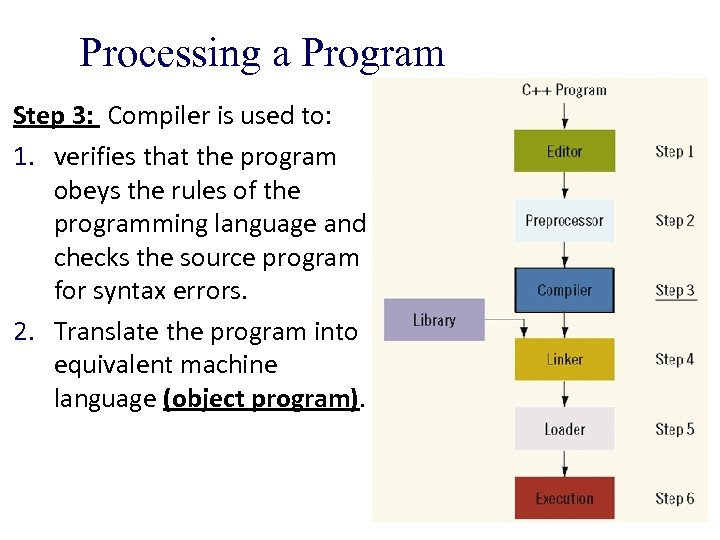Processing a Program Step 3: Compiler is used to: 1. verifies that the program obeys the rules of the programming language and checks the source program for syntax errors. 2. Translate the program into equivalent machine language (object program).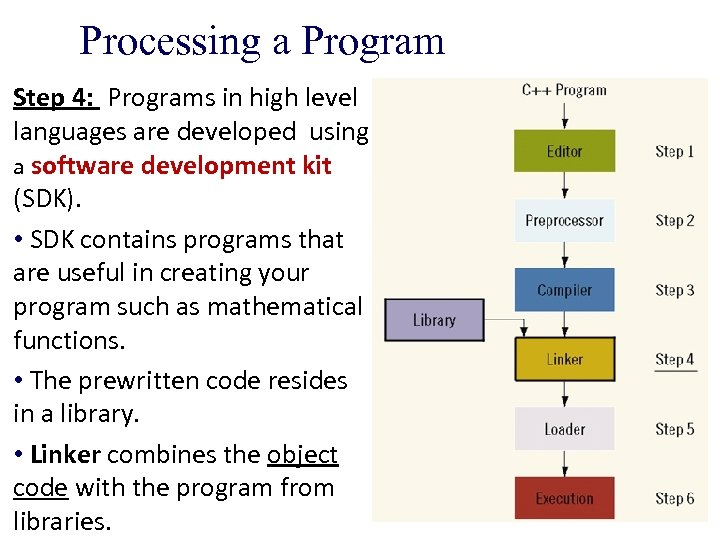Processing a Program Step 4: Programs in high level languages are developed using a software development kit (SDK). • SDK contains programs that are useful in creating your program such as mathematical functions. • The prewritten code resides in a library. • Linker combines the object code with the program from libraries.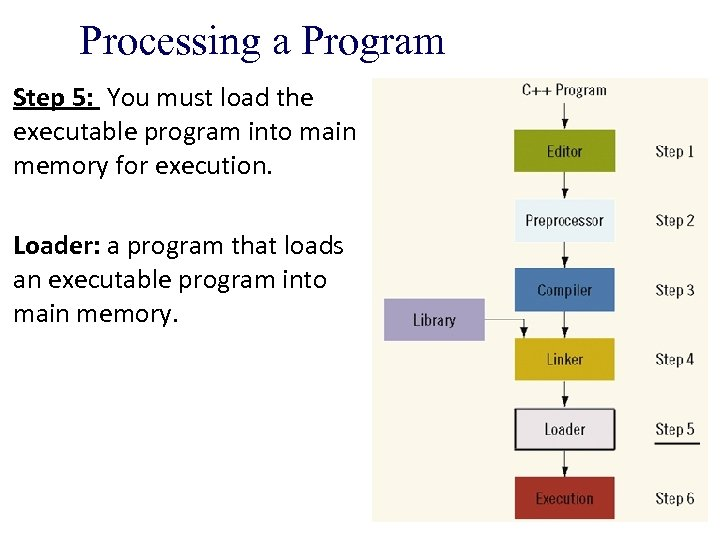Processing a Program Step 5: You must load the executable program into main memory for execution. Loader: a program that loads an executable program into main memory.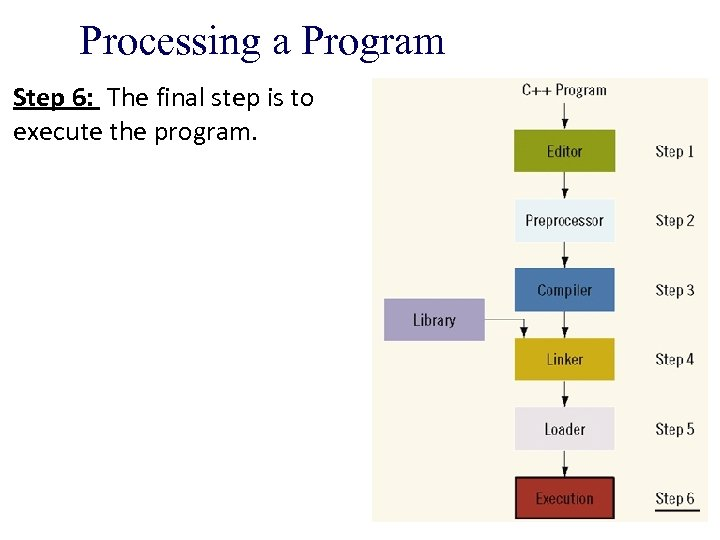Processing a Program Step 6: The final step is to execute the program.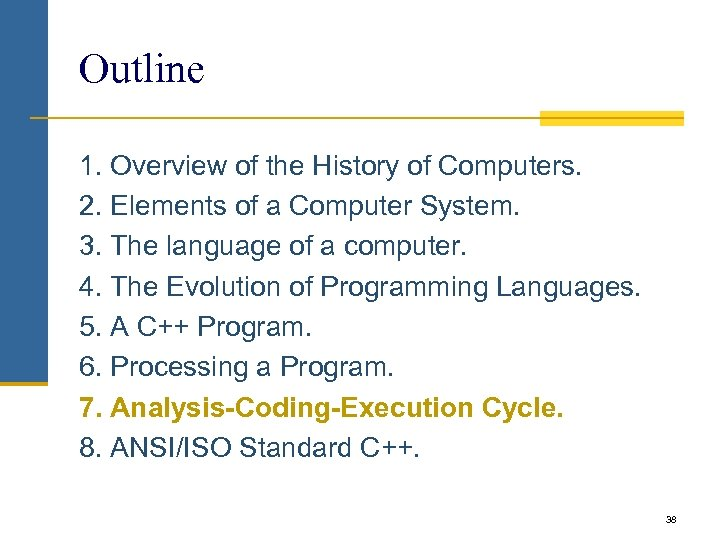Outline 1. Overview of the History of Computers. 2. Elements of a Computer System. 3. The language of a computer. 4. The Evolution of Programming Languages. 5. A C++ Program. 6. Processing a Program. 7. Analysis-Coding-Execution Cycle. 8. ANSI/ISO Standard C++. 38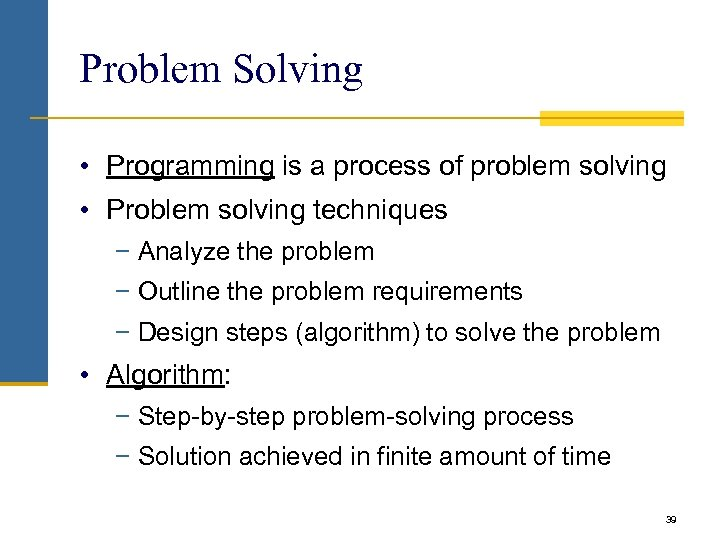Problem Solving • Programming is a process of problem solving • Problem solving techniques − Analyze the problem − Outline the problem requirements − Design steps (algorithm) to solve the problem • Algorithm: − Step-by-step problem-solving process − Solution achieved in finite amount of time 39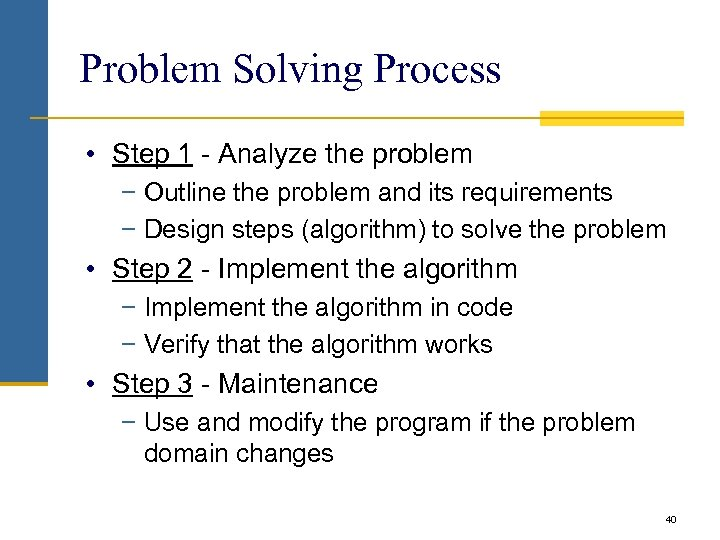Problem Solving Process • Step 1 - Analyze the problem − Outline the problem and its requirements − Design steps (algorithm) to solve the problem • Step 2 - Implement the algorithm − Implement the algorithm in code − Verify that the algorithm works • Step 3 - Maintenance − Use and modify the program if the problem domain changes 40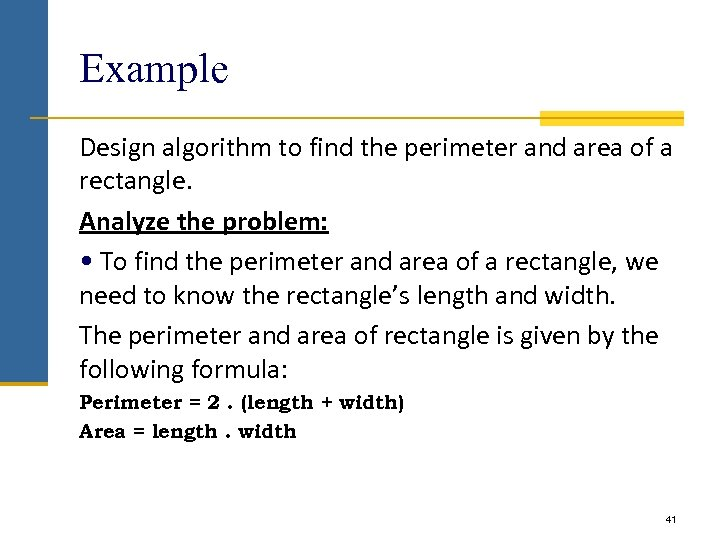Example Design algorithm to find the perimeter and area of a rectangle. Analyze the problem: • To find the perimeter and area of a rectangle, we need to know the rectangle’s length and width. The perimeter and area of rectangle is given by the following formula: Perimeter = 2. (length + width) Area = length. width 41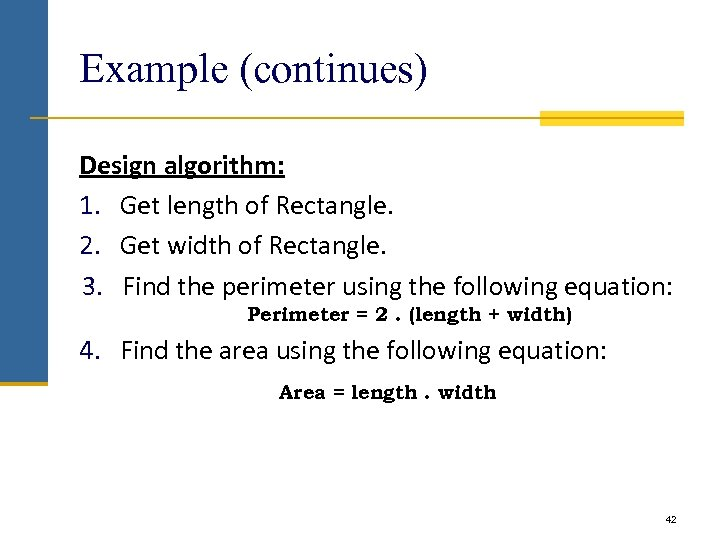Example (continues) Design algorithm: 1. Get length of Rectangle. 2. Get width of Rectangle. 3. Find the perimeter using the following equation: Perimeter = 2. (length + width) 4. Find the area using the following equation: Area = length. width 42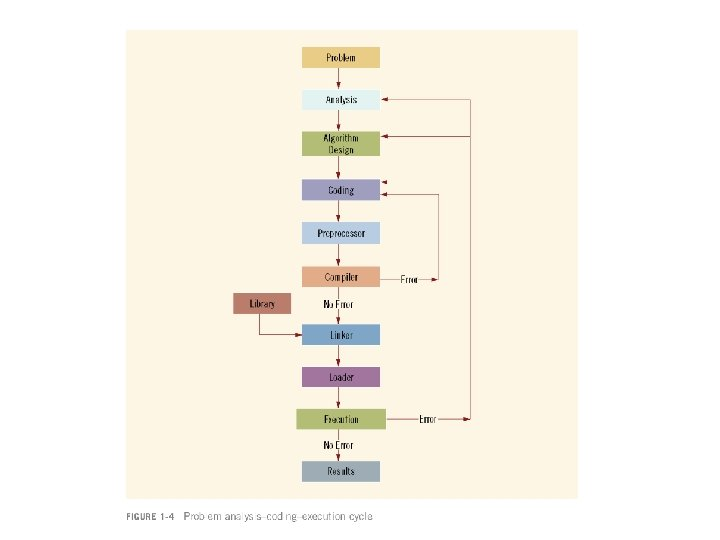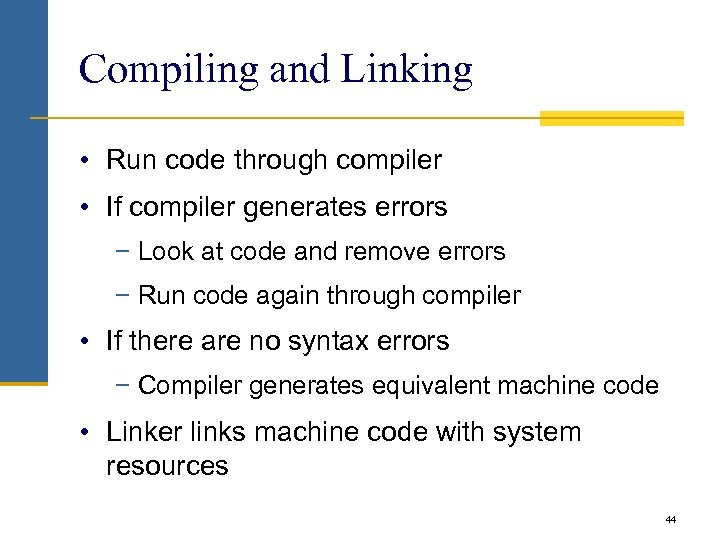Compiling and Linking • Run code through compiler • If compiler generates errors − Look at code and remove errors − Run code again through compiler • If there are no syntax errors − Compiler generates equivalent machine code • Linker links machine code with system resources 44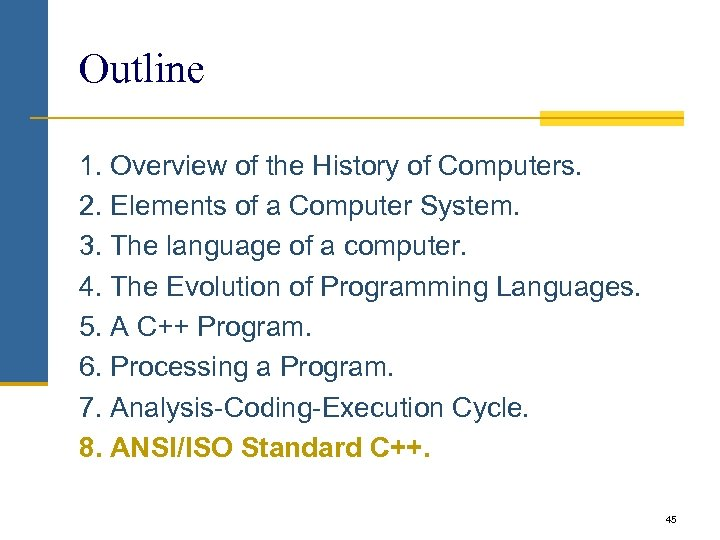Outline 1. Overview of the History of Computers. 2. Elements of a Computer System. 3. The language of a computer. 4. The Evolution of Programming Languages. 5. A C++ Program. 6. Processing a Program. 7. Analysis-Coding-Execution Cycle. 8. ANSI/ISO Standard C++. 45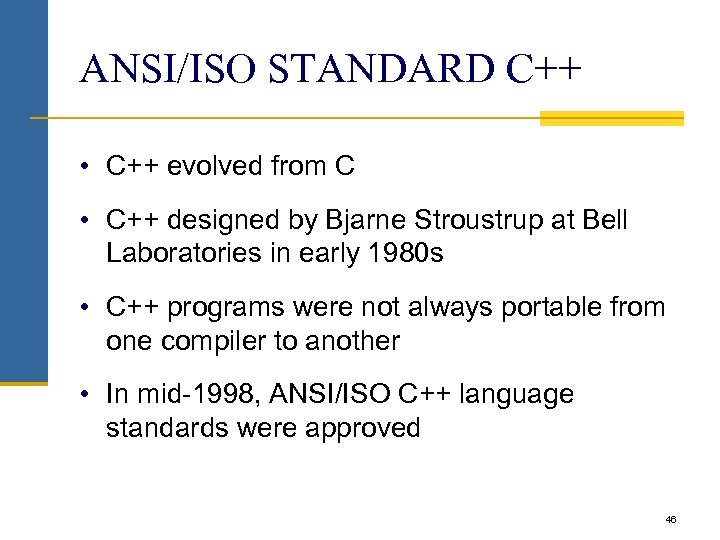ANSI/ISO STANDARD C++ • C++ evolved from C • C++ designed by Bjarne Stroustrup at Bell Laboratories in early 1980 s • C++ programs were not always portable from one compiler to another • In mid-1998, ANSI/ISO C++ language standards were approved 46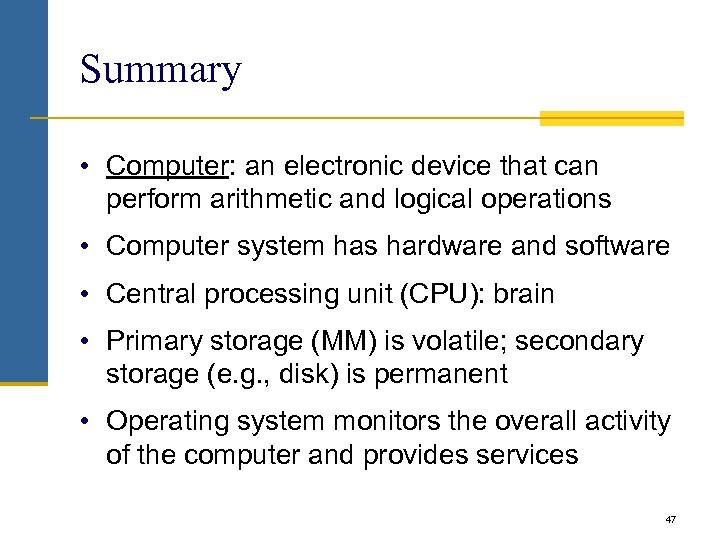Summary • Computer: an electronic device that can perform arithmetic and logical operations • Computer system has hardware and software • Central processing unit (CPU): brain • Primary storage (MM) is volatile; secondary storage (e. g. , disk) is permanent • Operating system monitors the overall activity of the computer and provides services 47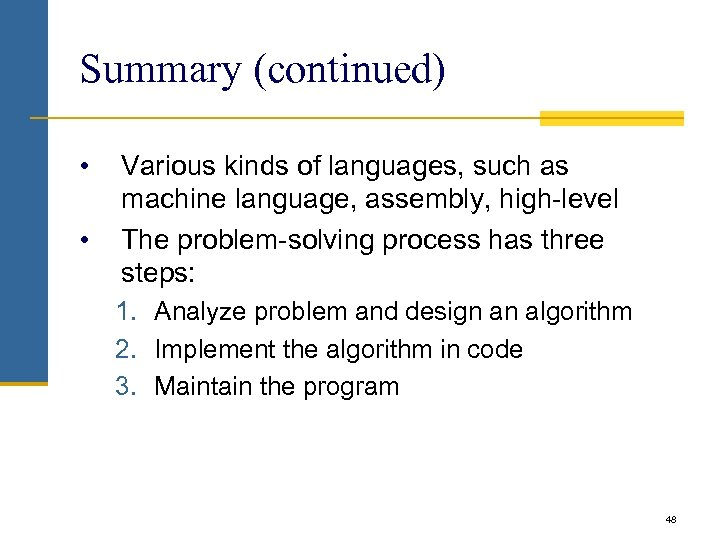Summary (continued) • • Various kinds of languages, such as machine language, assembly, high-level The problem-solving process has three steps: 1. Analyze problem and design an algorithm 2. Implement the algorithm in code 3. Maintain the program 48